# Useful Tips on How to Use Equation Editor in Word

##### Ivan Cook

• Filed to: PDF Tips

In Microsoft software, the equation editor is used for writing equations in a mathematical format. Authors of mathematics and engineering documents may need to include certain formulae within the content of their files. Most symbols used in formulae aren’t the conventional symbols found on keyboards generally. Hence they need to have full knowledge about how to use the equation editor efficiently. Basically, the equation editor is not difficult to use, one can save substantial time if shortcut keys are used to write the equations. This article will represent you how to use equation editor in Word.

## Part 1: Equation Editor for Word 2016

Equation editors are programs used to typeset or format mathematical formulae. Equation Editors come in handy when working on documents that contain technical details—usually mathematics-related. Equation Editors are also used in generating raster images for web pages and presentations among other types of works. In other words, an Equation Editor is a program that helps insert equations/formulae into documents.

Tip 1: We can go to the "Insert" tab, and from there to have the equation drop-down list. There are a few built-in functions and a bunch of options here. Some basic math symbols, fractions, some superscripts, subscripts, radicals, and so on are provided.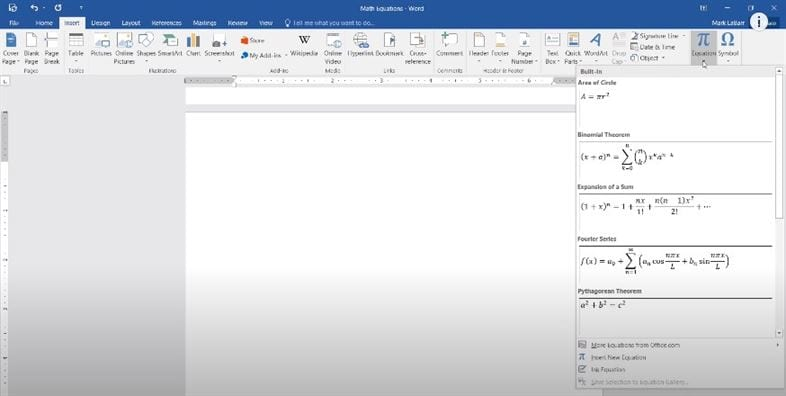Tip 2: Another thing we can do is that we can insert the equation just write. Word can pick it up quite well and works quite nicely. You even have a few options on changing its style. You can give it a more professional style or just give it a normal format.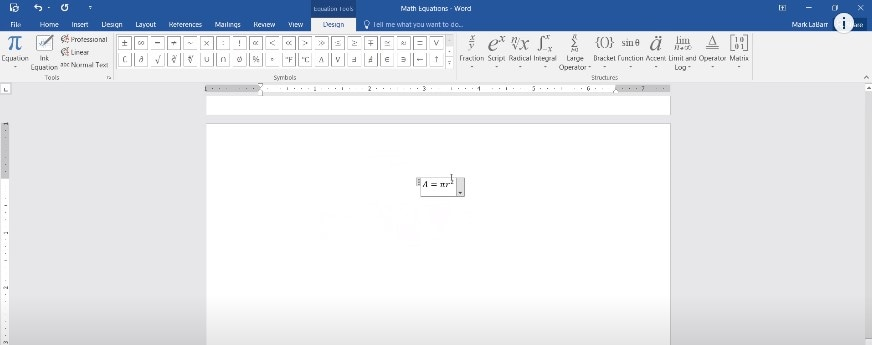## Part 2: 4 Tips on How to Get Equation Editor in Word 2007

Word 2007's method of entering equations from the keyboard and building up equations is more natural, intuitive, and efficient than earlier approaches to typesetting equations. Word's internal representation of the equations resembles mathematics and is much more readable. It uses the AutoCorrect feature of MS Word to do some of the formattings, and it introduces a concept of "Linear" vs. "Professional" formats. The linear format is what you might use in a programming language to write mathematics: e.g., y = (a*x+b)/(x^2+1). This part will show you how to get equation editor in Word 2007.

Tip 1: To insert an equation in a Word 2007 document, click on the "Insert" menu/tab to see the "Symbols" ribbon. In this section, choose "Equation". You will now see the equation tools. You can also press the "Alt+=" on your keyboard to get it.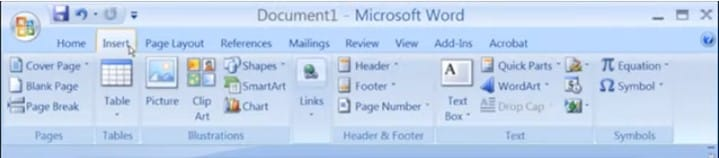Tip 2: In the "Tools" section, you have access to the "Equation" gallery and can select "Professional", "Linear" or "Normal Text" modes. Professional format is rendered two-dimensional math, as you would write on paper or a blackboard. The linear format is the equation editor's internal format. Normal text is for non-mathematical text annotations within an equation.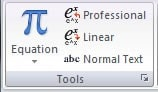Tip 3: The "Symbols" section contains commonly-used mathematical symbols. You can use the two scroll buttons to see more or click the 'more' icon to view all of the "Basic Math" symbols. Then click on "Basic Math" to see other sections of symbols, such as basic math, greek letters, letter-like symbols, operators, arrows, and so on.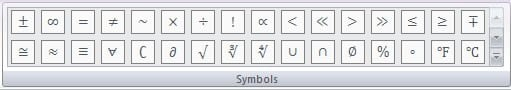Tip 4: The "Structures" section contains the formatting tools you will use to create equations. Like a template with symbols and placeholders for you to place your mathematical content into.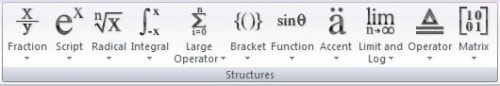## Part 3: How to Use Equation Editor in Word 2003

In Word, place the insertion point where you want the math symbol or template. From the Insert menu, select Object. The Object dialog box appears. Then click OK. The Equation Editor and Equation toolbar appear.

Step 1: Launch Word and open the equation editor from the "Insert" menu. Place the insertion point where you want the math symbol or template, then press the "Object" button from the "Insert" menu. An object dialog box appears. Click "OK". Then the equation editor and equation toolbar appear.

Step 2: From the "Equation" toolbar, click the symbol or template that you want to insert from the options.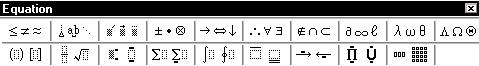Step 3: Once you select a symbol or template it appears in the work area in the equation editor. From this, you can modify your symbols. If you insert a template, you can create an equation.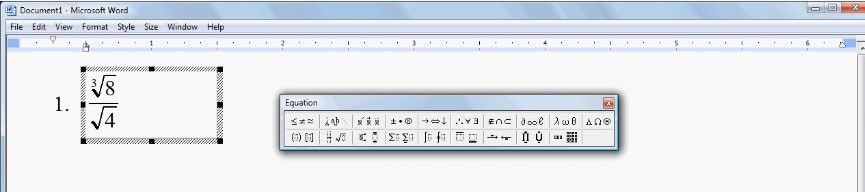## Part 4: Steps on Using Equation Editor in WPS

Step 1: Launch the program and open a document. Place cursor where you want to insert the fraction number. Click the "Equation" icon in the "Insert" tab to open the equation editor dialogue box.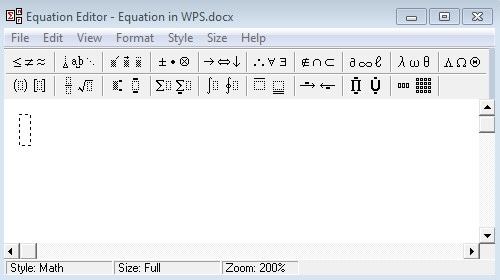Step 2: Select "Fraction and Radical" templates from the equation editor toolbar. Then choose a fraction style from the drop-down list and enter the fraction number in the editor.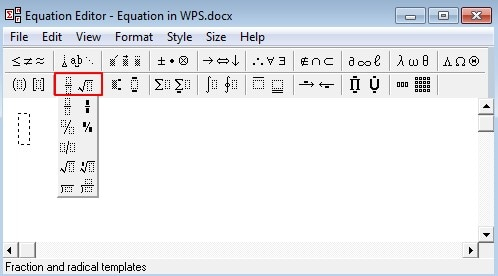Step 3: After you finish entering the numbers, close the equation editor to insert the fraction into your document.

## Conclusion

You've read the entire article now, so you might already have a clear thought on how to use equation editor in Word. Word document is a widely used format. It often is seen as the most convenient format for collecting and editing information. However, more and more people prefer to use PDF format these years, for the reason that it cannot be changed. Then the question comes, what if you need to use an equation editor for your PDF file? Or, you want to experience more functions to edit PDF? Great news! Now you have the chance to try one of the best PDF software: PDFelement (or PDFelement for Mac). As a specialized PDF software, it not only can edit PDF. Its convert function can help you to convert your PDF document to Word, and other formats. So that you can easily use equation editor in Word. PDFelement provides a quick and simple solution for you to deal with your PDF document.

#### Ivan Cook

chief Editor

> PDF Tips > Useful Tips on How to Use Equation Editor in Word